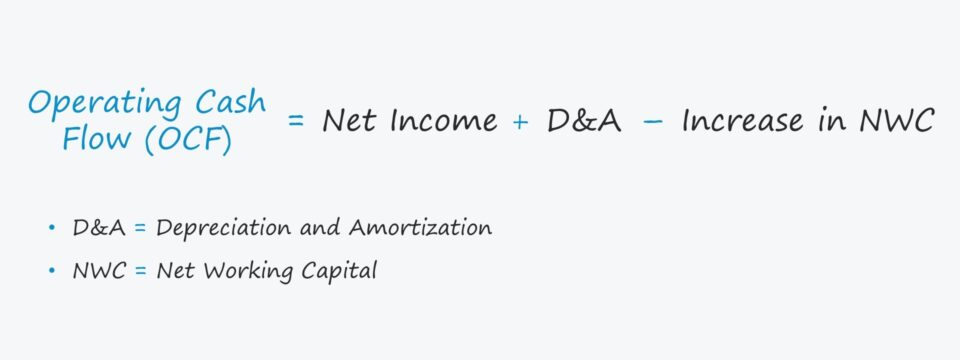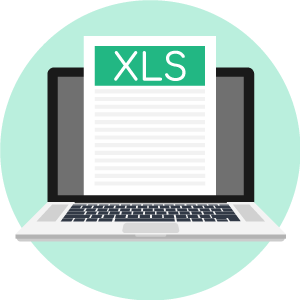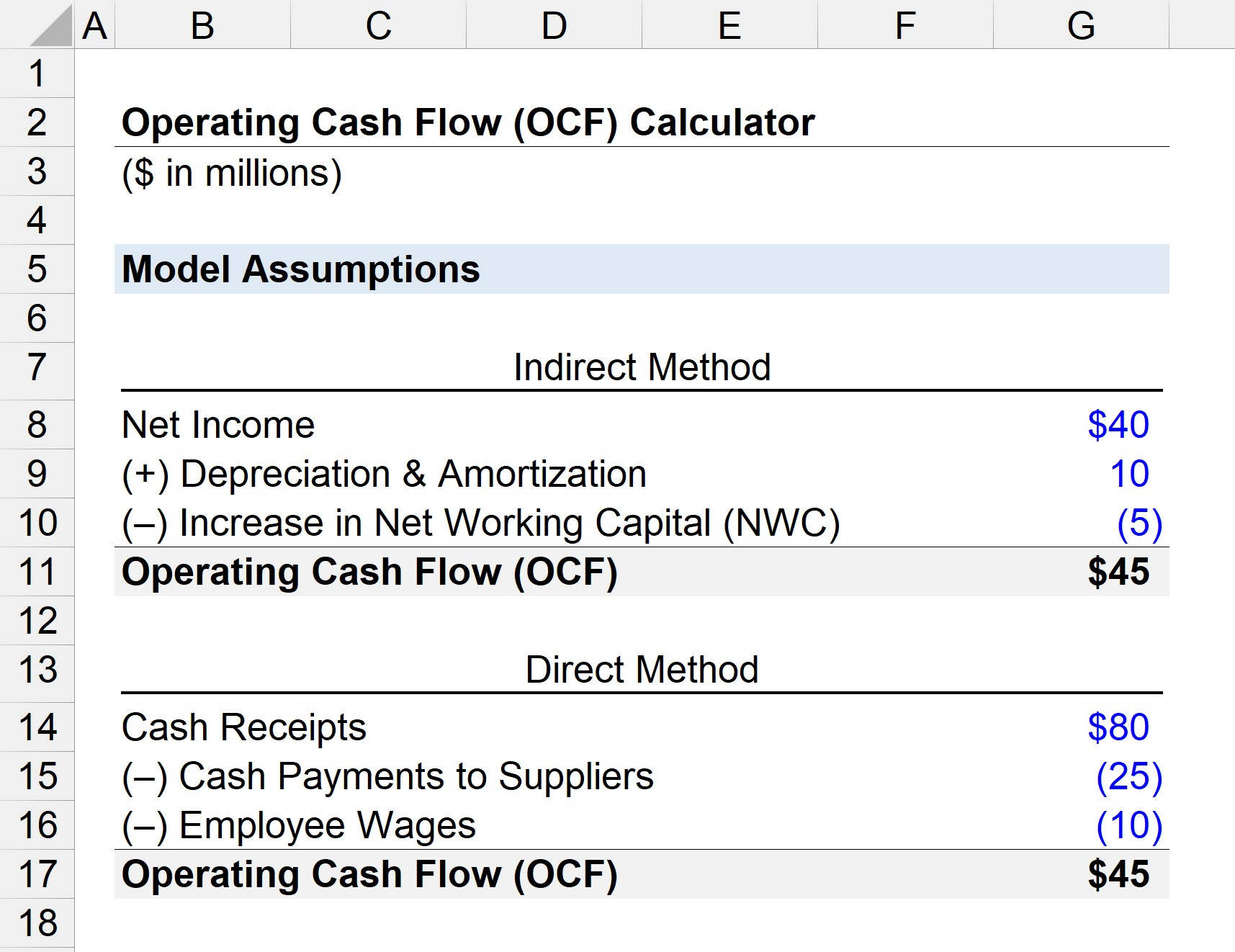# Operating Cash Flow (OCF)

Guide to Understanding Operating Cash Flow (OCF)• What does operating cash flow (OCF) measure?
• What is the operating cash flow (OCF) formula?
• How does the direct method of calculating operating cash flow (OCF) differ from the indirect method?
• How is operating cash flow (OCF) different from free cash flow (FCF)?

## How to Calculate Operating Cash Flow (OCF)

OCF, short for “operating cash flow,” refers to the net amount of cash brought in by a company’s day-to-day operations.

The income statement is reported per accounting standards established by U.S. GAAP, which does not typically reflect a company’s actual liquidity (i.e. cash on hand).

Hence, the cash flow statement (CFS) is necessary to understand the real cash inflows / (outflows) from operating, investing, and financing activities.

The CFS starts with the “Cash Flow from Operating Activities” section, which calculates a company’s operating cash flow (OCF) in a specified period of time.

In a scenario with positive OCF, the company’s operations generate adequate cash to meet its reinvestment needs, e.g. working capital and capital expenditures (CapEx).

But in the latter case with negative OCF, the company must seek external financing sources to meet its reinvestment spending needs, e.g. via equity and debt issuances.

The cash flow statement (CFS) can be presented under two methods — the indirect or the direct method:

1. Indirect Method: The beginning line item is net income, which is adjusted for non-cash items (e.g. D&A) and changes in working capital to arrive at cash flow from operations.
2. Direct Method – Instead of starting with net income, the direct method utilizes cash accounting to track the cash received from customers and paid out to third parties (e.g. suppliers, vendors).

## Operating Cash Flow (OCF) Formula — Indirect Method

Under the indirect method — the more common approach in the U.S. — the CFS’s top-line item is the accrual-based net income.

1. The starting line item, net income (the “bottom line”), is first adjusted by adding back non-cash expenses (e.g. such D&A, stock-based compensation).
2. Then, other adjustments are made for the changes in working capital.
3. The CFO section converts the accrual-based net income metric by adjusting it for non-cash items (e.g. depreciation, amortization) and changes in net working capital (NWC).
4. Once those adjustments have been made, the resulting line item is the “Cash Flow from Operating Activities”, i.e. the the operating cash flow (OCF).

If we consolidate all the steps up to this point, we come up with the following formula:

###### OCF Formula — Indirect Method
• Operating Cash Flow (OCF) = Net Income + Depreciation & Amortization – Increase in NWC

If OCF deviates substantially from net income, it implies further analysis is necessary to understand the underlying factors that are causing the difference.

The relationship between changes in working capital items and their respective cash flow impacts are as follows:

• Increase in Working Capital Asset → Cash Outflow (”Use”)
• Decrease in Working Capital Asset → Cash Inflow (”Source”)
• Increase in Working Capital Liability → Cash Inflow (”Source”)
• Decrease in Working Capital Liability → Cash Outflow (”Use”)
Working Capital Assets Working Capital Liabilities
• Inventories

For instance, if OCF is much lower than net income due to rising accounts receivable (A/R) — i.e. the sales in which customers paid on credit rather than cash — the company might have to reconsider how it collects cash payments from customers.

## Operating Cash Flow (OCF) Formula — Direct Method

The less prevalent approach to calculate OCF is the direct method, which utilizes cash accounting to track the movement of cash during a specified period.

Compared to the indirect method, the direct method is simpler, as the formula consists of subtracting cash operating expenses from cash revenue.

###### OCF Formula — Direct Method

Operating Cash Flow (OCF) = Cash Revenue – Operating Expenses Paid in Cash

To emphasis, only cash revenue and cash operating expenses are included under the direct method.

## Operating Cash Flow (OCF) vs Free Cash Flow (FCF)

OCF differs from FCF because the calculation of FCF includes CapEx, unlike OCF.

Operating cash flow (OCF) and free cash flow (FCF) are both metrics used to assess the financial stability of a company, typically to determine if the cash generated is enough to meet its spending needs.

While there are several variations of calculating free cash flow (FCF) — namely, free cash flow to firm (FCFF) and free cash flow to equity (FCFE) — the simplest formula subtracts capital expenditures (CapEx) from cash from operations (CFO).

###### Free Cash Flow (FCF) Formula
• Free Cash Flow (FCF) = Cash from Operations (CFO) – CapEx

The distinction between FCF and CFO is that FCF also deducts CapEx, as its a major cash outflow that is a core part of a company’s ability to produce cash flows.

For either metric, the higher the amount, the better off the company is (and vice versa), but FCF goes an extra step by considering CapEx.

## Operating Cash Flow (OCF) Excel Calculator

We’ll now move to a modeling exercise, which you can access by filling out the form below.Submitting ...

## OCF Example Calculation

Suppose that a company has the following financials:

• Net Income = \$40 million
• Depreciation & Amortization = \$10 million
• Increase in Net Working Capital (NWC) = –\$5 million

Our starting point is net income, which is pulled from the income statement.

The D&A amount is a non-cash add back (i.e. the real cash outflow, CapEx, already occurred in the initial period of purchase), so it has a positive impact on cash flow.

An increase in NWC reflects that there is more cash tied up in operations, thereby the cash flow decreases.

If we enter those assumptions into the OCF formula under the indirect method, we arrive at \$45 million as our illustrative company’s OCF.

• Operating Cash Flow (OCF) = \$40 million + \$10 million – \$5 million
• OCF = \$45 million

In the next part of our modeling exercise, we’ll calculate OCF using the direct method.

Here, we’ll use the following assumptions:

• Cash Receipts = \$80 million
• Cash Payments to Suppliers = –\$25 million
• Employee Wages = –\$10 million

Cash receipts refer to the cash payments received from customers, and the two cash operating expenses (i.e. cash reductions) consist of the following:

1. Supplier Payments
2. Employee Wages

Upon entering the assumptions into our OCF formula under the direct method, our company’s OCF is \$45 million.

• Operating Cash Flow (OCF) = \$80 million – \$25 million – \$10 million
• OCF = \$45 millionStep-by-Step Online Course

#### Everything You Need To Master Financial Modeling

Enroll in The Premium Package: Learn Financial Statement Modeling, DCF, M&A, LBO and Comps. The same training program used at top investment banks.Inline FeedbacksLearn Financial Modeling Online

Everything you need to master financial and valuation modeling: 3-Statement Modeling, DCF, Comps, M&A and LBO.

X

The Wall Street Prep Quicklesson Series

7 Free Financial Modeling Lessons

Get instant access to video lessons taught by experienced investment bankers. Learn financial statement modeling, DCF, M&A, LBO, Comps and Excel shortcuts.